# The HPQUANTSELECT Procedure

#### More Statistics for Parameter Estimates

Let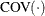denote the covariance function matrix of a random vector. Then, the sparsity-function estimates of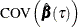is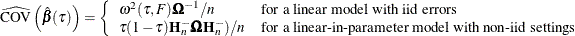where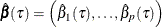is the vector of the parameter estimates.

If you specify the CLB option in the MODEL statement, PROC HPQUANTSELECT outputs the standard error, confidence limits, t value, and Pr >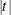probability for each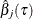in the parameter estimates table. Table 59.5 summarizes these statistics for.

Table 59.5: More Statistics forStatistic

Definition

Standard error: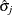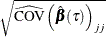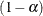% confidence limits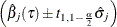t value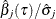Pr >probability

p-value of the t value

Here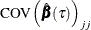is the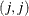element of, and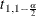denotes the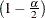-level student’s t score with 1 degree of freedom.